# Whole Number Division (F)

In this GCSE Maths quiz you will learn a few hints and tips that will make division a little bit easier! Use the divisibility tests when you are applying the ‘common sense’ check to your answers, and you can even use them to impress your friends!

• Only even numbers are divisible by 2.
• A number is divisible by 3 if the sum of the digits is in the three times table.
• If the last 2 digits of a number are in the four times table then it is divisible by 4.
• Only numbers ending in 0 or 5 are divisible by 5.
• For a number to be divisible by 6, it has to be divisible by 2 AND 3.
• The divisibility test for 7 is the hardest – take the last digit, multiply it by 2 and subtract from the other digits. If the answer is zero or in the seven times table then it is divisible by 7.
• Only the last three digits of a number need to be divisible by 8.
• A number is divisible by 9 if the sum of the digits is in the nine times table.

Knowing these divisibility tests can even help you with long division. If you have to divide by a number greater than 10, see if you can break it into its factors. Then perform repeated division until you reach an answer. So, if you are asked to divide a number by 24, you could divide it by 4, and then divide the result by 6. This is much easier, and less likely to result in mistakes, than the traditional long division method!

Question 1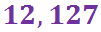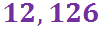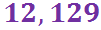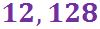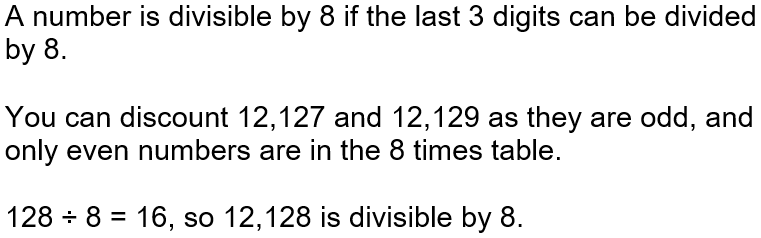Question 2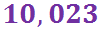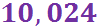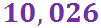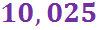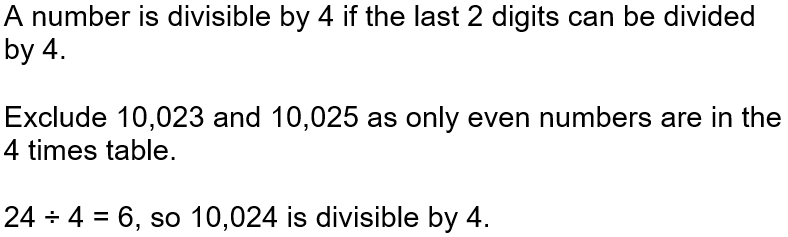Question 3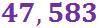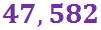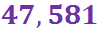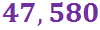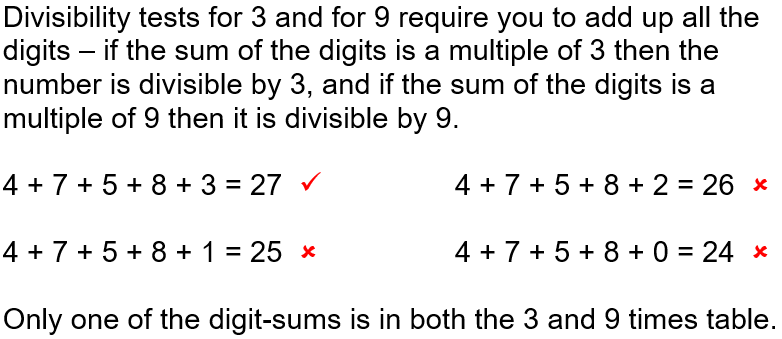Question 4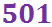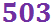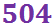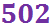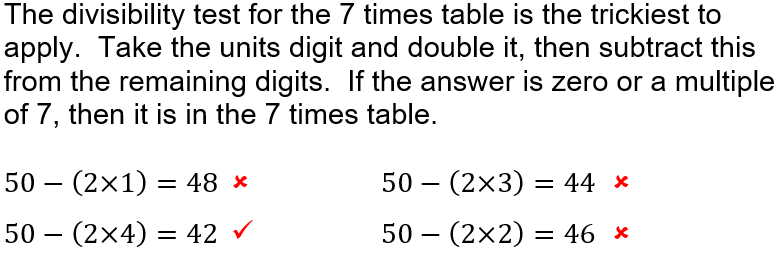Question 5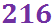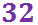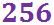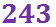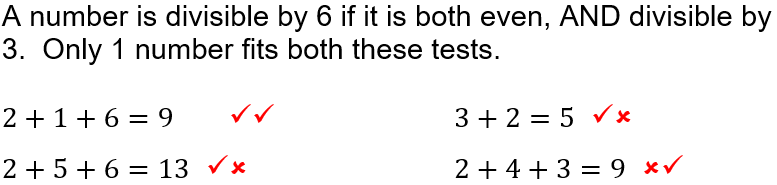Question 6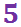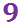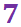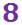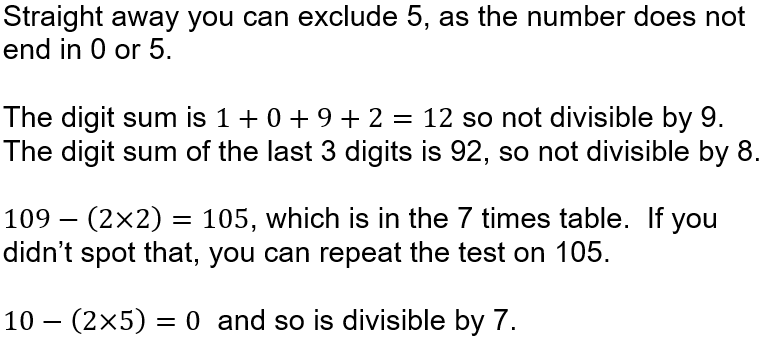Question 7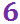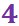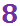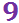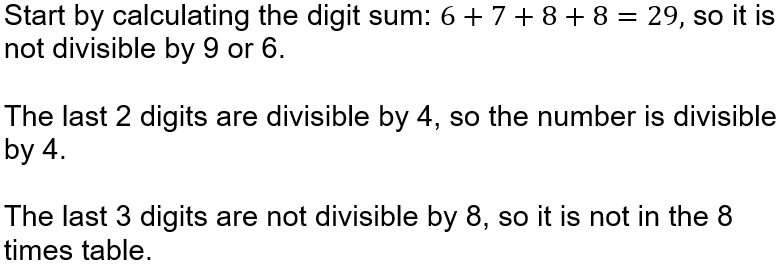Question 8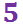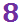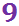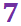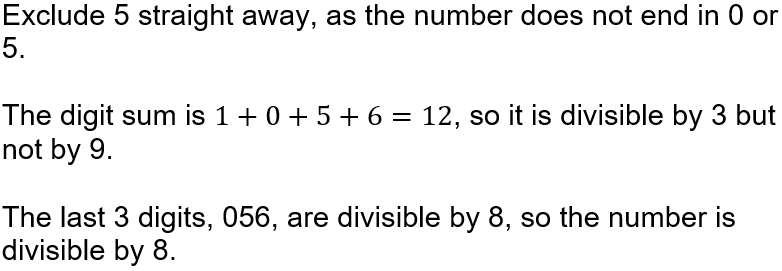Question 9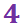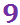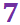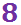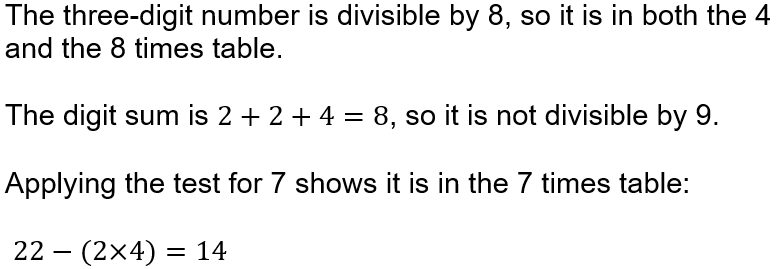Question 10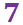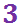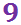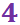Author:  Sally Thompson